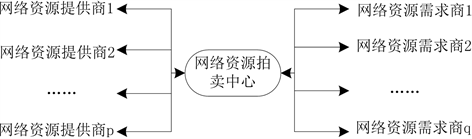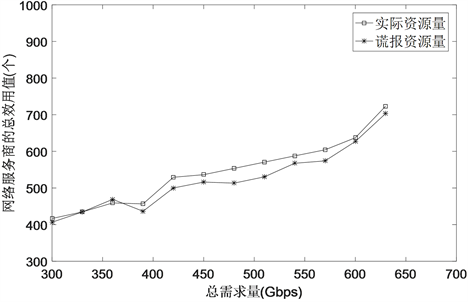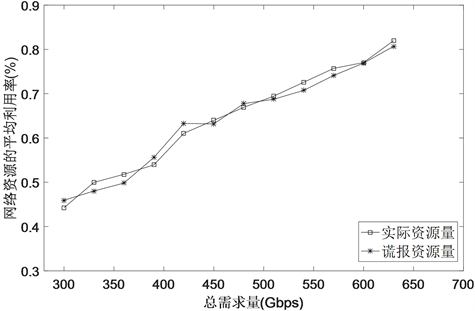# 主配网一体化环境下基于拍卖的网络服务商选择算法Auction-Based Network Service Provider Selection Algorithm in the Main Distribution Network Integration Environment

DOI: 10.12677/CSA.2020.109166, PDF, HTML, XML, 下载: 313  浏览: 434  国家科技经费支持

Abstract: In order to solve the problem of low network resource utilization and low resource allocation effi-ciency in the main distribution network integration environment, this paper models the network resource allocation problem as the three-party game problem, consisting of network resource provider, network resource demander and the resource auction center, and then proposes a resource allocation model. In order to maximize the social benefits of multiple network service providers and multiple network resource demanders, an auction-based network service provider selection algorithm is proposed, which proposes social welfare maximization strategy based on VCG auction theory. In the simulation experiment part, by comparing with the existing resource allocation algorithm, the resource allocation algorithm proposed in this paper achieves good results in terms of the average utilization rate and total utility value of network resources.

1. 引言

2. 问题描述和资源分配模型

2.1. 问题描述

${C}_{{\text{NSP}}_{i}}=\left\{\begin{array}{ll}0\hfill & \text{\hspace{0.17em}}\text{\hspace{0.17em}}\text{\hspace{0.17em}}\text{\hspace{0.17em}}\text{\hspace{0.17em}}{y}_{{\text{NSP}}_{i}}^{j}=0\hfill \\ {\sum }_{j=1}^{m}\left({f}_{{\text{NSP}}_{i}}^{j}+{y}_{{\text{NSP}}_{i}}^{j}×{u}_{{\text{NSP}}_{i}}^{j}\right)\hfill & 0<{y}_{{\text{NSP}}_{i}}^{j}\le c{p}_{{\text{NSP}}_{i}}^{j}\hfill \end{array}$ (1)

2.2. 资源分配模型Figure 1. Resource allocation model

3. 资源分配算法

3.1. 目标函数

${X}^{*}=\mathrm{arg}\underset{\mathrm{X}}{\mathrm{min}}\underset{i}{\sum }\underset{j}{\sum }\left({\lambda }_{j}{f}_{{\text{NSP}}_{i}}^{j}+{y}_{{\text{NSP}}_{i}}^{j}×{u}_{{\text{NSP}}_{i}}^{j}\right)$ (2)

${\lambda }_{j}=\left\{\begin{array}{cc}1& {y}_{{\text{NSP}}_{i}}^{j}>0\\ 0& {y}_{{\text{NSP}}_{i}}^{j}=0\end{array}$ (3)

${y}_{{\text{NSP}}_{i}}^{j}\le c{p}_{{\text{NSP}}_{i}}^{j}$ (4)

$\underset{k,l}{\sum }{y}_{{\text{NSD}}_{k}}^{l}=\underset{i,j}{\sum }{y}_{{\text{NSP}}_{i}}^{j}$ (5)

3.2. NSP的开销

${C}_{i}\left({x}_{i},{\theta }_{i}\right)=\left({\lambda }_{i}{f}_{{\text{CPE}}_{i}}^{j}+{y}_{{\text{CPE}}_{i}}^{j}×{u}_{{\text{CPE}}_{i}}^{j}\right)$ (6)

3.3. NSP的报酬

$\begin{array}{l}{R}_{i}=\left[\underset{x}{\mathrm{min}}\underset{{y}_{{\text{NSP}}_{t}}^{j}\le c{p}_{{\text{NSP}}_{t}}^{j},{\text{NSP}}_{t}\in {I}_{\text{NSP}}\i}{\sum }\left(\alpha \stackrel{^}{{f}_{{\text{NSP}}_{t}}^{j}}+\stackrel{^}{{u}_{{\text{NSP}}_{t}}^{j}}{x}_{t}^{j}\right)\right]\\ \text{\hspace{0.17em}}\text{\hspace{0.17em}}\text{\hspace{0.17em}}\text{\hspace{0.17em}}\text{\hspace{0.17em}}\text{\hspace{0.17em}}-\left[\underset{{\text{NSP}}_{t}\in {I}_{\text{NSP}},-i}{\sum }\left({\alpha }^{*}\stackrel{^}{{f}_{{\text{NSP}}_{t}}^{j}}+\stackrel{^}{{u}_{{\text{NSP}}_{t}}^{j}}{\stackrel{^}{{x}_{t}^{j}}}^{*}\right)\right]\end{array}$ (7)

3.4. NSP的惩罚函数

${P}_{i}={R}_{i}\left\{{x}_{i}^{j*}\ge \stackrel{¯}{{x}_{i}^{j}}\right\}$ (8)

3.5. NSP的效用函数

NSP的效用是指NSP将网络销售给NSD时获得的效用。本文定义NSP的效用函数为公式(9)，其中， ${P}_{i}$ 表示NSPi为了多销售网络而作假遭到的惩罚，NSPi上报给网络资源拍卖中心的网络提供量采用 ${\theta }_{i}=\left(\stackrel{^}{{f}_{{\text{NSP}}_{i}}^{j}},\stackrel{^}{{u}_{{\text{NSP}}_{i}}^{j}},\stackrel{^}{c{p}_{{\text{NSP}}_{i}}^{j}}\right)$ 表示。NSPi分配它的 ${\theta }_{i}$ 网络 ${x}_{i}\in x$ 给NSD的开销采用 ${C}_{i}\left({x}_{i},{\theta }_{i}\right)$ 表示。NSPi销售它的 ${\theta }_{i}$ 网络 ${x}_{i}\in x$ 给NSD后获得的报酬采用 ${R}_{i}$ 表示。

${U}_{i}\left({x}_{i},{R}_{i},{\theta }_{i}\right)={R}_{i}-{C}_{i}\left({x}_{i},{\theta }_{i}\right)-{P}_{i}$ (9)

3.6. 基于拍卖的网络服务商选择算法Table 1. Network service provider selection algorithm based on auction

4. 仿真

4.1. 环境

4.2. 评价指标

${R}_{\text{NSP}}=\frac{{y}_{{\text{NSP}}_{i}}^{c}+{y}_{{\text{NSP}}_{i}}^{e}}{c{p}_{{\text{NSP}}_{i}}^{c}+c{p}_{{\text{NSP}}_{i}}^{e}}$ (10)

${U}_{\text{NSP}}={\sum }_{i=1}^{N}{U}_{i}\left({x}_{i},{R}_{i},{\theta }_{i}\right)$ (11)

4.3. 验证资源分配算法的有效性Figure 2. Comparison of the total utility valuesFigure 3. Comparison of the average utilization rate of network resources

5. 总结

  汪磊, 魏丽芳, 王克谦, 等. 主配网调控一体化图形平台设计[J]. 电力系统及其自动化学报, 2012, 24(1): 142-146.  曹军威, 万宇鑫, 涂国煜, 等. 智能电网信息系统体系结构研究[J]. 计算机学报, 2013, 36(1): 143-167.  石悦, 邱雪松, 郭少勇, 等. 基于改进遗传算法的电力光传输网规划方法[J]. 通信学报, 2016, 37(1): 116-122.  Shi, Y., Qiu, X.-S. and Guo, S.Y. (2015) Genetic Algorithm-Based Redundancy Optimization Method for Smart Grid Communication Network. China Communications, 12, 73-84. https://doi.org/10.1109/CC.2015.7224708  Yu, R., Zhong, W.F., Xie, S.L., Zhang, Y. and Zhang, Y. (2016) QoS Differential Scheduling in Cognitive-Radio- Based Smart Grid Networks: An Adaptive Dynamic Program-ming Approach. IEEE Transactions on Neural Networks and Learning Systems, 27, 435-443. https://doi.org/10.1109/TNNLS.2015.2411673  孟洛明, 孙康, 韦磊, 等. 一种面向电力无线专网的虚拟资源优化分配机制[J]. 电子与信息学报, 2017, 39(7): 1711-1718.  Matias, J., Garay, J., Toledo, N., Unzilla, J. and Jacob, E. (2015) Toward an SDN-Enabled NFV Architecture. IEEE Communications Magazine, 53, 187-193. https://doi.org/10.1109/MCOM.2015.7081093  Liang, C.C. and Yu, F.R. (2015) Wireless Network Virtualization: A Survey, Some Research Issues and Challenges. IEEE Communications Surveys & Tutorials, 17, 358-380. https://doi.org/10.1109/COMST.2014.2352118  Correa, E.S., Fletscher, L.A. and Botero, J.F. (2015) Virtual Data Center Embedding: A Survey. IEEE Latin America Transactions, 13, 1661-1670. https://doi.org/10.1109/TLA.2015.7112029  Guan, X.J., Choi, B.Y. and Song, S. (2015) Energy Effi-cient Virtual Network Embedding for Green Data Centers Using Data Center Topology and Future Migration. Computer Communications, 69, 50-59. https://doi.org/10.1016/j.comcom.2015.05.003  Melo, M. and Sargento, S. (2015) Optimal Virtual Network Embedding: Energy Aware Formulation. Computer Networks, 91, 184-195. https://doi.org/10.1016/j.comnet.2015.08.011  Vickrey, W.W. (1961) Counter Speculation, Auctions, and Competitive Sealed Tenders. Journal of Finance, 16, 8-36. https://doi.org/10.1111/j.1540-6261.1961.tb02789.x  Bae, J., Beigman, E., Berry, R.A., Honig, M.L. and Vohra, R. (2008) Sequential Bandwidth and Power Auctions for Distributed Spectrum Sharing. IEEE Journal on Selected Areas in Communications, 26, 1193-1203. https://doi.org/10.1109/JSAC.2008.080916  Guan, X.J., Choi, B.Y. and Song, S. (2015) Energy Effi-cient Virtual Network Embedding for Green Data Centers Using Data Center Topology and Future Migration. Computer Communications, 69, 50-59. https://doi.org/10.1016/j.comcom.2015.05.003  Xia, M., Koehler, G.J. and Whinston, A.B. (2004) Pricing Combinatorial Auctions. European Journal of Operational Research, 154, 251-270. https://doi.org/10.1016/S0377-2217(02)00678-1